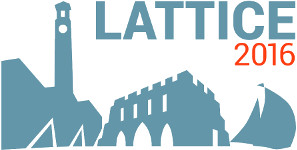#The 34th International Symposium on Lattice Field Theory (Lattice 2016)

Jul 24 – 30, 2016
Highfield Campus, University of Southampton
Europe/London timezone

## Isovector Axial Charge with Current Improvement

Jul 26, 2016, 8:00 PM
1h
Highfield Campus, University of Southampton

#### Highfield Campus, University of Southampton

Highfield Campus, Southampton SO17 1BJ, UK

### Speaker

Dr YIBO Yang (university of Kentucky)

### Description

We employ dimension 4 operators to improve the local vector and axial vector currents, as the leading order approximation of the lattice conserved current, and then calculate the nucleon iso-vector axial coupling $g^3_A$ using overlap valence on Domain Wall Fermion sea. Using the equality of $g^3_A$ from $A_i$ and $A_4$ components of the axial-vector current as a normalization condition in addition to axial Ward identity, we find two to three percent increase of $g^3_A$ towards the experimental value. The excited state contamination has been taken into account with three time separations between the source and sink. The improved axial charges $g_A^{IM}(24I)=1.188(7)$, $g_A^{IM}(32I)=1.177(9)$ are obtained on $24^3\times 64$ and $32^3\times 64$ lattices at the unitary point where the pion masses are $330$ MeV and $300$ MeV respectively.

### Primary author

Dr Jian Liang (University of Kentucky)

### Co-authors

Prof. Andrei Alexandru (The George Washington University) Prof. Keh-Fei Liu (University of Kentucky) Raza Sufian (University of Kentucky, United States) Prof. Terrence Draper (University of Kentucky) Dr YIBO Yang (university of Kentucky)

### Presentation materials

There are no materials yet.